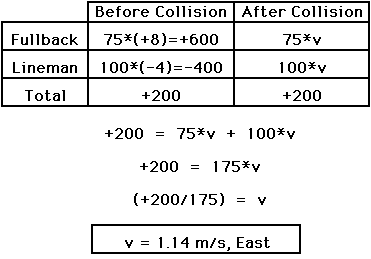Momentum and Its Conservation - Lesson 2 - The Law of Momentum Conservation

# Using Equations as a Guide to Thinking

The three problems on the previous page illustrate how the law of momentum conservation can be used to solve problems in which the after-collision velocity of an object is predicted based on mass and velocity information. There are similar practice problems (with accompanying solutions) lower on this page that are worth the practice. However, let's first take a more qualitative approach to some collision problems. The questions that follow provide a real test of your conceptual understanding of momentum conservation in collisions.

Suppose that you have joined NASA and are enjoying your first space walk. You are outside the space shuttle when your fellow astronaut of approximately equal mass is moving towards you at 2 m/s (with respect to the shuttle). If she collides with you and holds onto you, then how fast (with respect to the shuttle do you both move after the collision?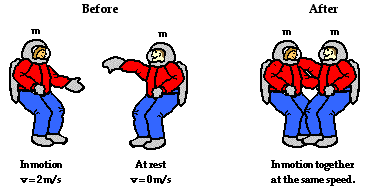This problem could be solved in the usual manner with a momentum table; the variable m could be used for the mass of the astronauts or any random number could be used for the mass of the astronauts (provided each astronaut had the same mass). In the process of solving the problem, the mass would cancel out of the momentum conservation equation and the post-collision velocities could be determined. However, there is a moreconceptual means of solving this problem. In order for the momentum before the collision to be equal to the momentum after the collision, the after collision velocity must be smaller than the before collision velocity. How many times smaller must it be? By what factor must the velocity be decreased? Before the collision, the amount of mass in motion is m; after the collision, the amount of mass in motion is 2•m. The amount of mass in motion has doubled as the result of the collision. If the mass is increased by a factor of two, then the velocity must be decreased by a factor of 2. The before-collision velocity was 2 m/s so the after-collision velocity must be one-half this value: 1 m/s. Each astronaut is moving with a velocity of 1 m/s after the collision.The process of solving this problem involved using a conceptual understanding of the equation for momentum (p=m*v). This equation becomes a guide to thinking about how a change in one variable affects a change in another variable. The constant quantity in a collision is the momentum (momentum is conserved). For a constant momentum value, mass and velocity are inversely proportional. Thus, an increase in mass results in a decrease in velocity.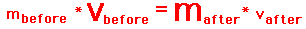A twofold increase in mass, results in a twofold decrease in velocity (the velocity is one-half its original value); a threefold increase in mass results in a threefold decrease in velocity (the velocity is one-third its original value); etc. Of course, it is instructive to point out that this form of problem solving is limited to situations in which one of the two objects is at rest before the collision and both objects move at the same speed after the collision. To further test your understanding of this type of quantitative reasoning, try the following two questions.

A large fish is in motion at 2 m/s when it encounters a smaller fish that is at rest. The large fish swallows the smaller fish and continues in motion at a reduced speed. If the large fish has three times the mass of the smaller fish, then what is the speed of the large fish (and the smaller fish) after the collision? Click the button to view answer.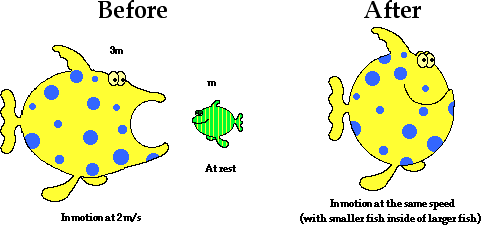A railroad diesel engine has five times the mass of a boxcar. A diesel coasts backwards along the track at 4 m/s and couples together with the boxcar (initially at rest). How fast do the two trains cars coast after they have coupled together? Click the button to view answer.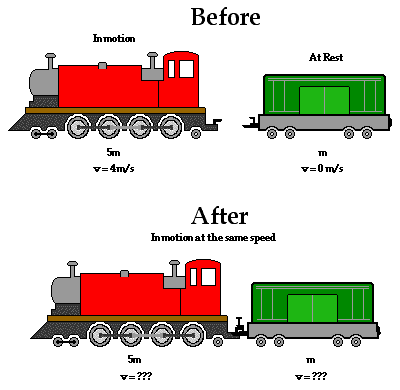### Watch It!

View the three inelastic collisions on a low-friction track, broadcasted from The Physics Classroom's YouTube channel.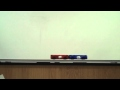m Collides with m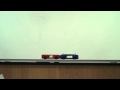m Collides with 2m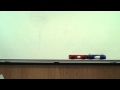m Collides with 3m

### We Would Like to Suggest ...Sometimes it isn't enough to just read about it. You have to interact with it! And that's exactly what you do when you use one of The Physics Classroom's Interactives. We would like to suggest that you combine the reading of this page with the use of our Fish Catch Interacative and/or our Collision Carts Ineractive. These Interactives can be found in the Physics Interactive section of our website and provide an interactive experience in analyzing the momentum of individual objects and systems of objects in collisions.

Visit:  Fish Catch  |  Collision Carts

Express your understanding of the concept and mathematics of momentum conservation by answering the following questions. Assume isolated systems and momentum conservation for each problem. Click the See Answer button to view answer. (If necessary, return to the instructional page on solving collision analysis problems.)

1. In a physics lab, 0.500-kg cart (Cart A) moving rightward with a speed of 92.8 cm/s collides with a 1.50-kg cart (Cart B) moving leftward with a speed of 21.6 cm/s. The two carts stick together and move as a single object after the collision. Determine the post-collision speed of the two carts.

See Solution

2. A 25.0-gram bullet enters a 2.35-kg watermelon and embeds itself in the melon. The melon is immediately set into motion with a speed of 3.82 m/s. The bullet remains lodged inside the melon. What was the entry speed of the bullet? (CAUTION: Be careful of the units on mass.)

See Solution

3. A 25.0-gram bullet enters a 2.35-kg watermelon with a speed of 217 m/s and exits the opposite side with a speed of 109 m/s. If the melon was originally at rest, then what speed will it have as the bullet leaves its opposite side? (CAUTION: Be careful of the units on mass.)

See Solution

4. In a physics lab, a 0.500-kg cart (Cart A) moving with a speed of 129 cm/s encounters a magnetic collision with a 1.50-kg cart (Cart B) that is initially at rest. The 0.500-kg cart rebounds with a speed of 45 cm/s in the opposite direction. Determine the post-collision speed of the 1.50-kg cart.

See Solution

5. A 3000-kg truck moving rightward with a speed of 5 km/hr collides head-on with a 1000-kg car moving leftward with a speed of 10 km/hr. The two vehicles stick together and move with the same velocity after the collision. Determine the post-collision velocity of the car and truck. (CAREFUL: Be cautious of the +/- sign on the velocity of the two vehicles.)

See Solution

6. During a goal-line stand, a 75-kg fullback moving eastward with a speed of 8 m/s collides head-on with a 100-kg lineman moving westward with a speed of 4 m/s. The two players collide and stick together, moving at the same velocity after the collision. Determine the post-collision velocity of the two players. (CAREFUL: Be cautious of the +/- sign on the velocity of the two players.)

See Solution

1. The problem can be solved using a momentum table: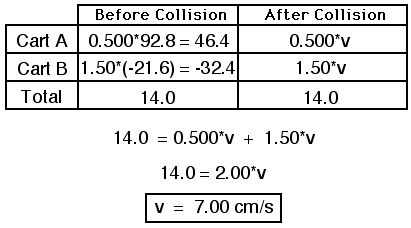2. The problem can be solved using a momentum table: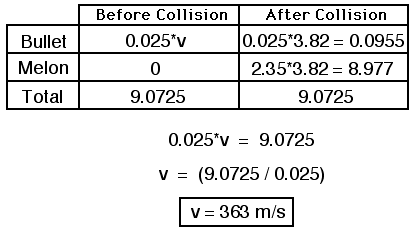3. The problem can be solved using a momentum table: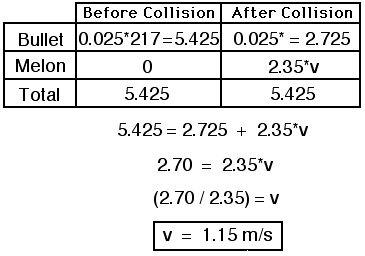4. The problem can be solved using a momentum table: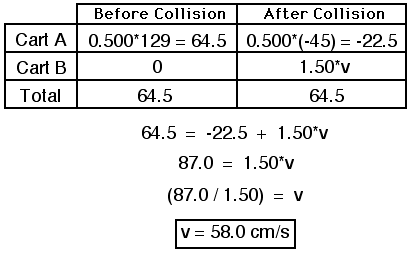5. The problem can be solved using a momentum table: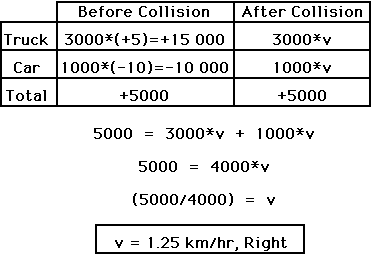6. The problem can be solved using a momentum table: